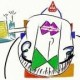On the ggplot2 mailing-list the following question was asked:

How to create a back-to-back bar chart with ggplot2?

For anyone who don’t know what I am talking about, have a look on a recent paper from the EU. I’d like to create plots like the graphs 5,6,18 in the paper.

An example graph from the above report is below:Let’s create the same graph in ggplot2.

I was not able to find the exact dataset used to plot the graph above, and used instead the Eurostat “EU27 trade by BEC product group since 1999” dataset which has a very similar data structure.

Access the subset used in this post in here.

 `> library(ggplot2)`
 ```> trade <- read.csv("trade.csv", header = TRUE, stringsAsFactors = FALSE)```

 ```> balance <- ddply(trade, .(Time), summarise, balance = sum(EXP - IMP))```

Convert data from wide format to long format for plotting.

 `> trade.m <- melt(trade, id.vars = c("BEC", "Time"))`
 ```> ggplot(trade.m, aes(Time)) + geom_bar(subset = .(variable == "EXP"), aes(y = value, fill = BEC), stat = "identity") + geom_bar(subset = .(variable == "IMP"), aes(y = -value, fill = BEC), stat = "identity") + xlab("") + scale_y_continuous("Export - Import", formatter = "comma")``````> labels <- gsub("20([0-9]{2})M([0-9]{2})", "\\2\n\\1", trade.m\$Time) > last_plot() + geom_line(data = balance, aes(Time, balance, group = 1), size = 1) + geom_hline(yintercept = 0, colour = "grey90") + scale_x_discrete(labels = labels)```The legend explanation (too large to fit on the plot):

• CAP Capital goods
• CNS Consumption goods
• CTR Consumption goods plus motor spirit and passenger motor cars
• INT Intermediate goods

There shouldn’t be any negative numbers on the y-axis, we need a custom formatter to convert these to positive.

 ```> commapos <- function(x, ...) { format(abs(x), big.mark = ",", trim = TRUE, scientific = FALSE, ...) }```
 `> last_plot() + scale_y_continuous(formatter = "commapos")`I have to agree with some of the posters on the mailing list, that this type of chart is not very easy to follow – it is impossible to compare individual values. Another useful way of showing information would be to use facets:

 ```> ggplot(trade.m, aes(Time)) + geom_bar(aes(y = value), stat = "identity") + facet_grid(variable ~ BEC) + scale_x_discrete("", labels = labels) + scale_y_continuous(formatter = "comma")```Or combine export and import values on one plot showing the comparative trend between the different goods categories.

 ```> ggplot(trade.m, aes(Time, colour = variable, group = variable)) + geom_line(aes(y = value)) + facet_grid(BEC ~ .) + scale_x_discrete("", labels = labels) + scale_y_continuous(formatter = "comma")```The same graph with varying scales across panels.

 `> last_plot() + facet_grid(BEC ~ ., scales = "free_y")`Update: As suggested in the comments it would be good to have total values added to the last plot.

In order to do so the totals are first calculated and then appended to the original dataframe.

 ```> total <- ddply(trade.m, .(Time, variable), summarise, value = sum(value), BEC = "Total") > total <- rbind(trade.m, total)```

Plotting the new dataframe is just a question of changing the source of the last plot.

 `> last_plot() %+% total`1.September 25, 2009 12:06 pm

gsub(“20([0-9]{2})M([0-9]{2})”, “\\2\n\\1”

I really need to learn me some regex 🙂

Maybe adding a “total” to the last facet_grid plot would be the best solution overall.

Thanks again

•September 25, 2009 3:53 pm

I updated the post with your suggestion.

2.March 25, 2010 5:12 pm

Is there a way to annotate the axes? In the example graph we have y axis annoted with “(million euro)”. I note that in your recreation in ggplot2, this annotation is ommited. Is it because it is easy to do, or the opposite? I’m trying to find something in the ggplot2 book, but with no luck.

•March 28, 2010 12:13 pm

Yes, it is very easy to change axis labels.

You could use

`xlab("(million euro)")`

or

`labs (x = "(million euro)")`

•March 30, 2010 11:30 am

You would need to resort to grid.text for annotating the axis. Try something like this:
`grid.text("Millions euros", x = unit(.05, "npc"), y = unit(.98, "npc"), hjust=0.4)`

3.March 30, 2012 2:06 am

These look great! Is there a way to apply the back to back bar chart for a value that is created from stat_summary? For example, if one is summing a variable by group and then wishes to plot each group (some are negative and some are positive) in a stack, how could one use the subset syntax?

•4.